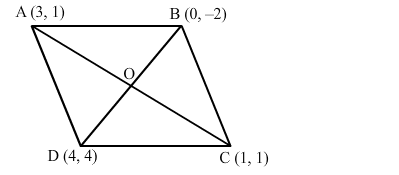# Show that the points A(3, 1), B(0, −2), C(1, 1) and D(4, 4)Question:

Show that the points A(3, 1), B(0, −2), C(1, 1) and D(4, 4) are the vertices of a parallelogram ABCD.

Solution:

The points are A(3, 1), B(0, −2), C(1, 1) and D(4, 4).
Join AC and BD, intersecting at O.We know that the diagonals of a parallelogram bisect each other.

Midpoint of $A C=\left(\frac{3+1}{2}, \frac{1+1}{2}\right)=\left(\frac{4}{2}, \frac{2}{2}\right)=(2,1)$

Midpoint of $B D=\left(\frac{0+4}{2}, \frac{-2+4}{2}\right)=\left(\frac{4}{2}, \frac{2}{2}\right)=(2,1)$

Thus, the diagonals AC and BD have the same midpoint.
Therefore, ABCD is a parallelogram.